﻿

### On homogeneous hypersurfaces in the manifold of quaternionic subalgebras of the Cayley algebra

#### Abstract

In this work  we consider a class of real hypersurfaces of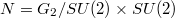$N=G_2 / SU(2) \times SU(2)$, the manifold of quaternionic subalgebras of the Cayley algebra. They are the  family of tubes centered at the maximal totally geodesic submanifolds of maximal rank of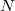$N$, which are (up to isomorphism)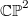$\mathbb{CP}^2$ and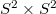$S^2 \times S^2$. We determine which of those tubes are homogeneous and for them we obtain the spectral decomposition of the shape operator. Moreover we show that the universal covering space of the focal set of$\mathbb{CP}^2$ is the sphere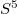$S^5$.

DOI Code: 10.1285/i15900932v21n2p119

Keywords: Manifold of quaternionic algebras; Hypersurfaces; Tubes; Shape operator; Jacobi operator

Classification: 53C30; 53C35; 53C42

Full Text: PDFThis work is licensed under a Creative Commons Attribuzione - Non commerciale - Non opere derivate 3.0 Italia License.